## Vector Calculator are math calculator to find vectors addition fast and easy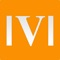# Vector Calculator Plus

by Nitrio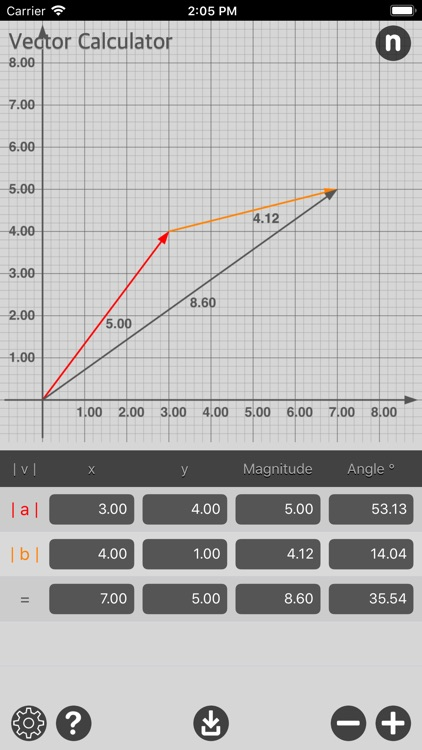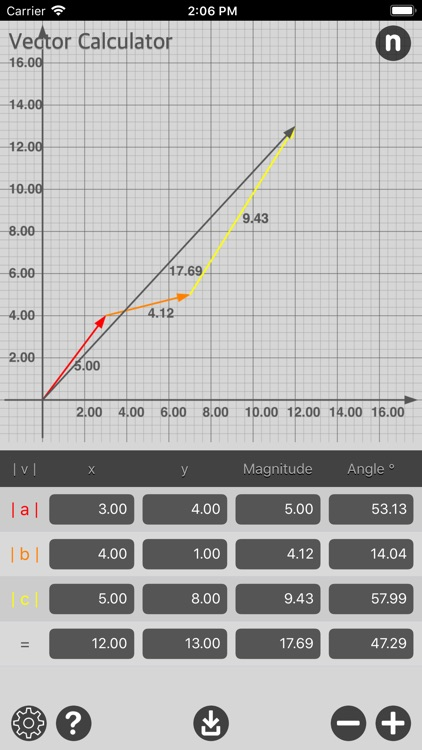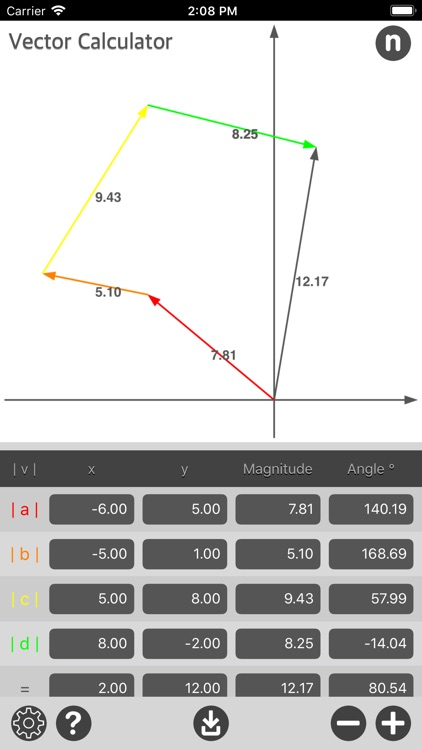Vector Calculator are math calculator to find vectors addition fast and easy.### App Details

Version
1.0
Rating
NA
Size
14Mb
Genre
Utilities Productivity
Last updated
March 30, 2019
Release date
March 29, 2019

### App Screenshots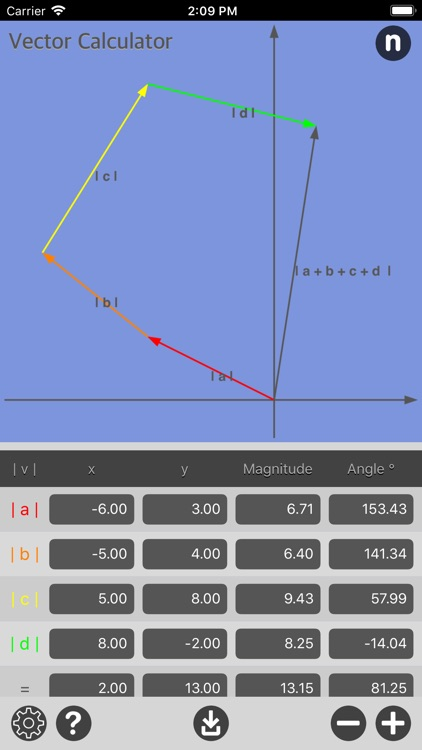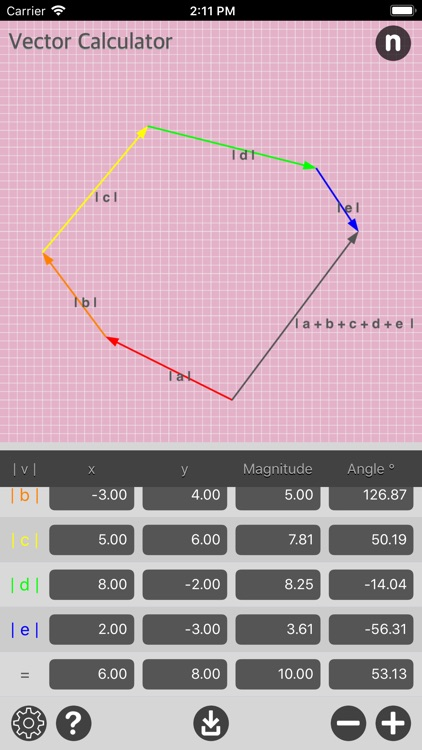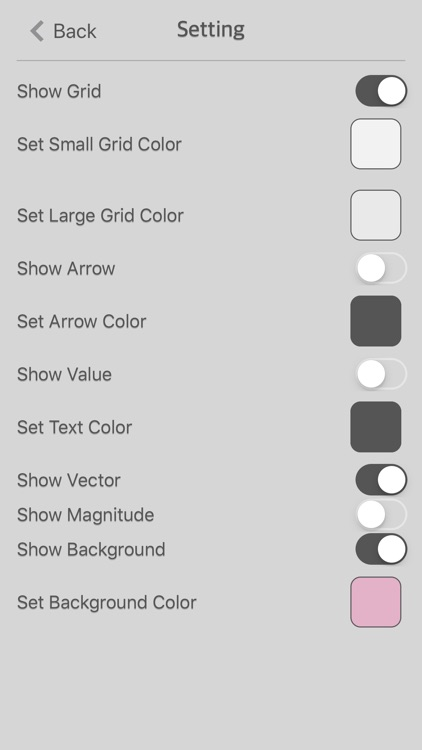### App Store Description

Vector Calculator are math calculator to find vectors addition fast and easy.
Vector Calculator give operation of adding two or more vectors together into a vector sum. The parallelogram law gives the rule for vector addition of two or more vectors.

Features:
- Instant vector calculation
- Add multiple vector by x, y, magnitude or angle
- Vector Image are generate upon calculation
- Save Vector Image into camera roll
- Option to show/hide Grid, Indicator, Label, Vector Label or Magnitude Label for Vector Graph
- Option to enable/disable Background for Vector Graph
- Option to set Background Colour for Vector Graph

A Vector has magnitude [size] and direction.
No matter which order you add them, you will get the same result.

Magnitude of a Vector:
The magnitude of a vector is shown by two vertical bars on either side of the vector

|a|

Example: add the vectors a = (2,3) and b = (4,5)

|a+b| = |a| + |b|

|a+b| = (2,3) + (4,5) = (2+4,3+5) = (6,8)

Common examples of vectors are force and velocity. Both force and velocity are in a particular direction. The magnitude of the vector would indicate the strength of the force or the speed associated with the velocity.

Vector Calculator is useful for math teacher, educator, or anyone who want to prepare vector math material for teaching.